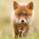‎1065‎ مشاهدة
1065
Request for JR, added fast MA's and conditions, modified the sl lines to display as lines and added option to show/hide them, modified shape to display arrows up/down
study(title="[RS]JR Moving Average System V1", shorttitle="[RS]JR.MAS.V1", overlay=true)
hide_sl_lines = input(true)
ma0 = sma(hl2, input(1))

tp = input(8)
ma1 = wma(open, tp)
ma2 = ema(open, tp)

//---   2nd pass
tf = input(2)
ma3 = wma(open, tf)
ma4 = ema(open, tf)
//---   2nd pass plot output not required.

plot(ma0, color=black)
plot(ma1, color=gray)
plot(ma2, color=silver)

condition = ma0 >= ma1 and ma1 >= ma2 ? (close >= open ? lime : green) :
ma0 <= ma1 and ma1 <= ma2 ? (close >= open ? red : maroon) :
ma0 >= ma3 and ma3 >= ma4 ? (close >= open ? lime : green) :
ma0 <= ma3 and ma3 <= ma4 ? (close >= open ? red : maroon) : (close >= open ? silver : gray)

barcolor(condition)

//  ||---   Crossovers
buyx1 = cross(ma0,ma1) and ma0 >= ma0 ? true : false
sellx1 = cross(ma0,ma1) and ma0 <= ma0 ? true : false
plotshape(sellx1, style=shape.arrowdown, color=maroon, location=location.abovebar)

//  ||---   SL lines
sltop = highest(max(max(high,ma0),max(ma1,ma2)), tp)
slbot = lowest(min(min(low,ma0),min(ma1,ma2)), tp)

tcol = sltop != sltop ? na : silver
bcol = slbot != slbot ? na : silver
plot(hide_sl_lines ? na : sltop, color=tcol, style=line)
plot(hide_sl_lines ? na : slbot, color=bcol, style=line)



## التعليقاتSince you have mad coding skills. If possible, could you code a simple indicator to help identify the % price is trading above a moving average. For example, the % the dax is trading above its 12 month mva. The period would be able to change depending on what period the user prefers. This would not be a signal indicator to buy or sell, just an indicator to help identify extremes when price trades away from a mva.
ردWhen rules are applied 2,8,2,8 Ichimoku gave no false signals for the chart above.
ردQuantitativeExhaustion
for ex., input(2.0, title="Mult") will allow user to enter decimal values for conversion line, lagging line and span/displacement.
ردQuantitativeExhaustion
opening above / under the cloud isnt enought as a filter?
it would filter these
ردRicardoSantos
Are you sure?

looking around March 6th I see a negative lead. I don't see how we could have gotten green candles.
ردRicardoSantos
Is that for the two Ichimoku signals alone? I'd wait on adding any other indications with Ichimoku.
ردx=input("240", title="Enter mins")

Above was mentioned by LazyBear as a way to include MTF inputs by the user for anytime frame.
ردQuantitativeExhaustion
yes only if chart time frame is lower then the signal timeframe not the other way around
ردRicardoSantos
ok. this should remove a bunch of false signals during sideways trades. I'll get back to this lower time frame hard signal / MTF idea.
رد